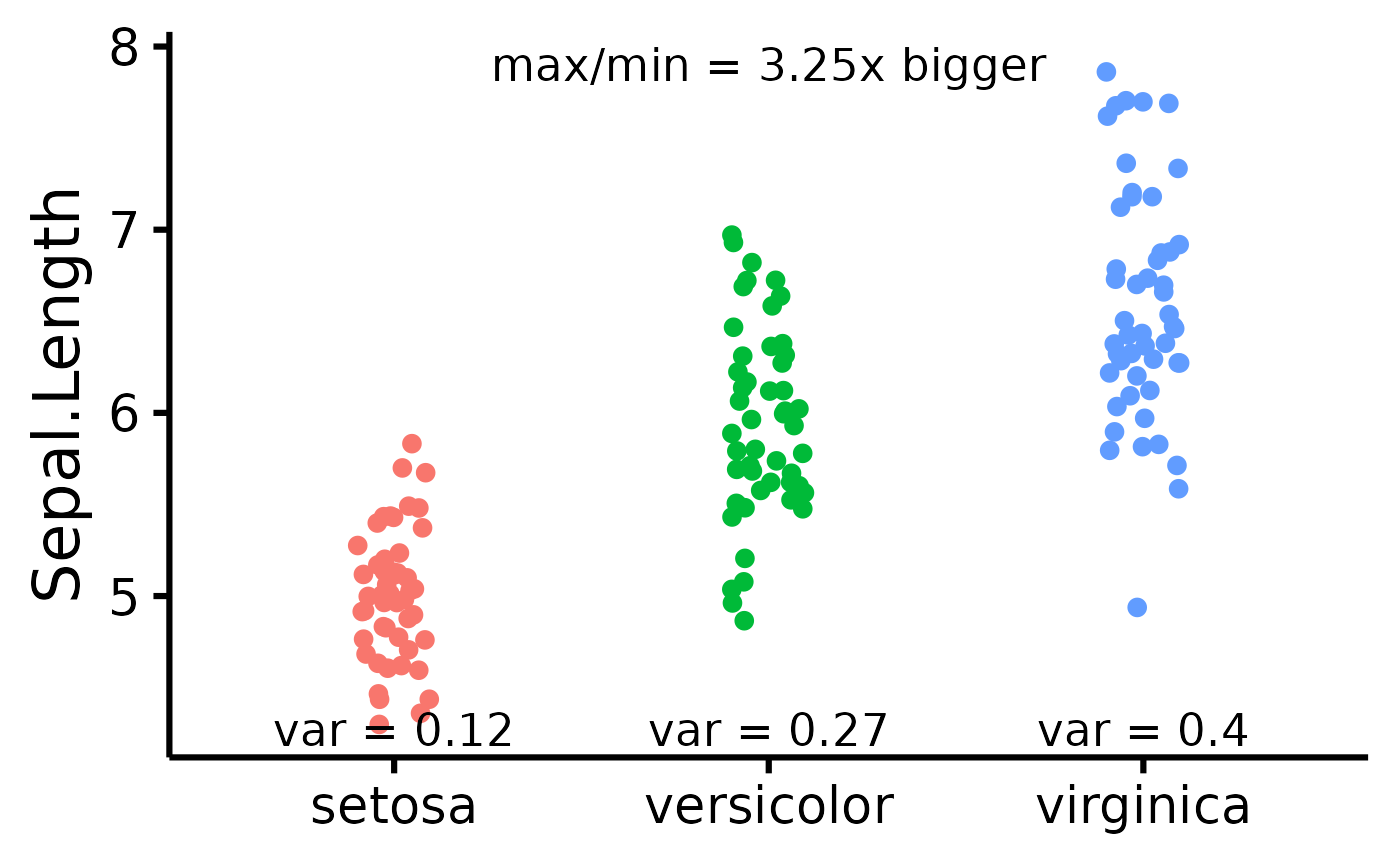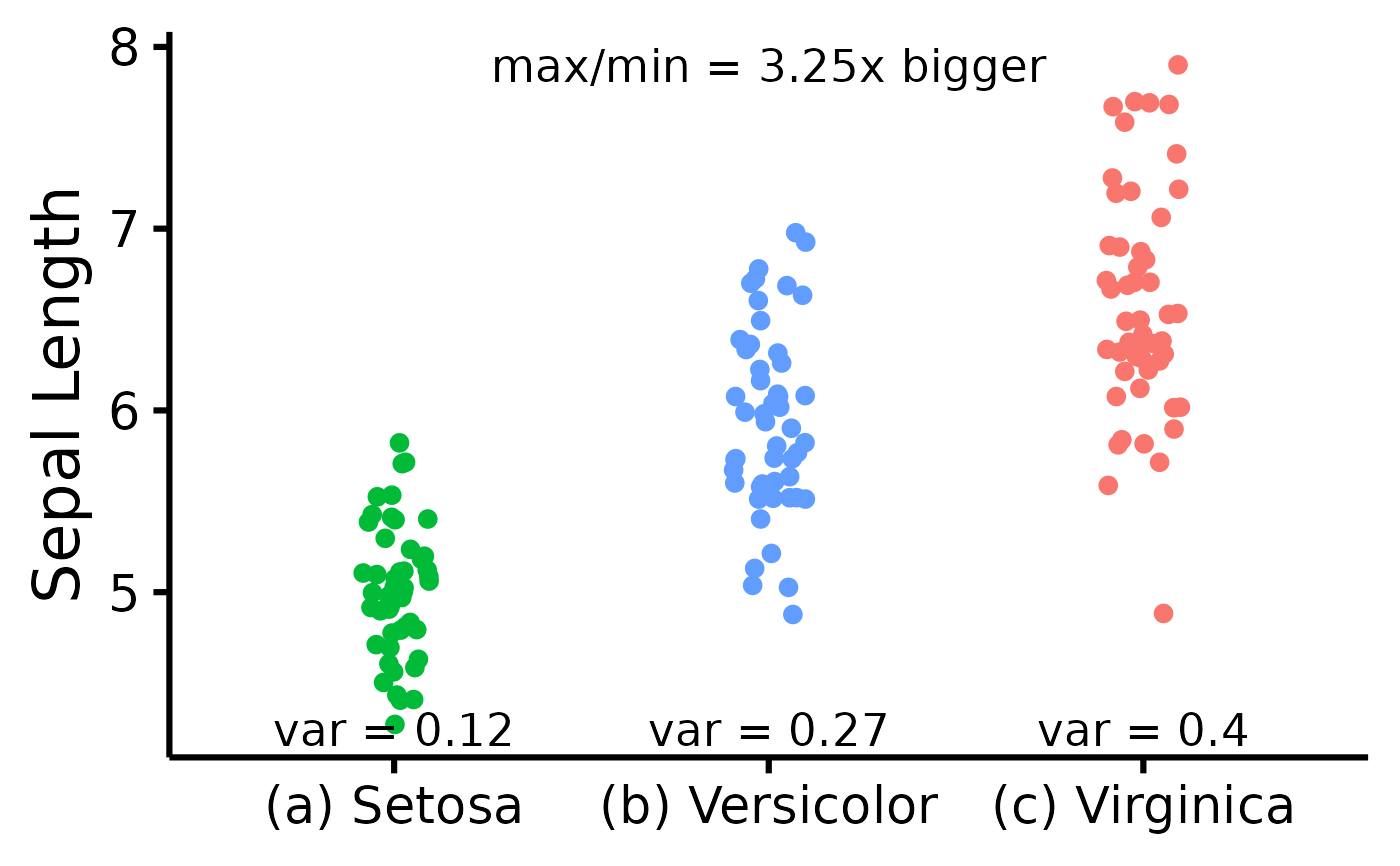Attempt to visualize variance per group.

## Usage

nice_varplot(
data,
variable,
group,
colours,
groups.labels,
grid = TRUE,
shapiro = FALSE,
ytitle = variable
)

## Arguments

data

The data frame

variable

The dependent variable to be plotted.

group

The group by which to plot the variable.

colours

Desired colours for the plot, if desired.

groups.labels

How to label the groups.

grid

Logical, whether to keep the default background grid or not. APA style suggests not using a grid in the background, though in this case some may find it useful to more easily estimate the slopes of the different groups.

shapiro

Logical, whether to include the p-value from the Shapiro-Wilk test on the plot.

ytitle

An optional y-axis label, if desired.

## Value

A scatter plot of class ggplot attempting to display the group variances. Also includes the max variance ratio (maximum variance divided by the minimum variance).

Other functions useful in assumption testing: nice_assumptions, nice_density, nice_normality, nice_qq, nice_var. Tutorial: https://rempsyc.remi-theriault.com/articles/assumptions

## Examples

# Make the basic plot
nice_varplot(
data = iris,
variable = "Sepal.Length",
group = "Species"
)# Further customization
nice_varplot(
data = iris,
variable = "Sepal.Length",
group = "Species",
colours = c(
"#00BA38",
"#619CFF",
"#F8766D"
),
ytitle = "Sepal Length",
groups.labels = c(
"(a) Setosa",
"(b) Versicolor",
"(c) Virginica"
)
)